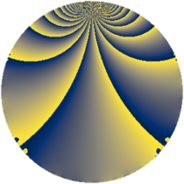# Properties

 Label 273.2.bhLevel $273$ Weight $2$ Character orbit 273.bh Rep. character $\chi_{273}(131,\cdot)$ Character field $\Q(\zeta_{6})$ Dimension $64$ Newform subspaces $1$ Sturm bound $74$ Trace bound $0$

# Related objects

## Defining parameters

 Level: $$N$$ $$=$$ $$273 = 3 \cdot 7 \cdot 13$$ Weight: $$k$$ $$=$$ $$2$$ Character orbit: $$[\chi]$$ $$=$$ 273.bh (of order $$6$$ and degree $$2$$) Character conductor: $$\operatorname{cond}(\chi)$$ $$=$$ $$21$$ Character field: $$\Q(\zeta_{6})$$ Newform subspaces: $$1$$ Sturm bound: $$74$$ Trace bound: $$0$$

## Dimensions

The following table gives the dimensions of various subspaces of $$M_{2}(273, [\chi])$$.

Total New Old
Modular forms 84 64 20
Cusp forms 68 64 4
Eisenstein series 16 0 16

## Trace form

 $$64q + 32q^{4} - 4q^{7} - 4q^{9} + O(q^{10})$$ $$64q + 32q^{4} - 4q^{7} - 4q^{9} - 12q^{10} - 30q^{12} + 12q^{15} - 16q^{16} - 10q^{18} + 10q^{21} - 8q^{22} + 36q^{24} - 36q^{25} - 20q^{28} - 22q^{30} + 12q^{31} + 36q^{36} - 36q^{40} + 48q^{42} - 32q^{43} - 6q^{45} - 48q^{46} + 36q^{49} - 16q^{51} - 54q^{54} - 8q^{57} - 12q^{58} + 16q^{60} - 72q^{61} - 86q^{63} - 48q^{64} - 78q^{66} + 32q^{67} - 4q^{70} + 62q^{72} + 48q^{73} + 48q^{75} - 20q^{78} - 64q^{79} + 28q^{81} + 72q^{82} - 18q^{84} + 64q^{85} + 60q^{87} + 44q^{88} + 8q^{91} - 38q^{93} + 72q^{94} + 66q^{96} - 68q^{99} + O(q^{100})$$

## Decomposition of $$S_{2}^{\mathrm{new}}(273, [\chi])$$ into newform subspaces

Label Dim. $$A$$ Field CM Traces $q$-expansion
$$a_2$$ $$a_3$$ $$a_5$$ $$a_7$$
273.2.bh.a $$64$$ $$2.180$$ None $$0$$ $$0$$ $$0$$ $$-4$$

## Decomposition of $$S_{2}^{\mathrm{old}}(273, [\chi])$$ into lower level spaces

$$S_{2}^{\mathrm{old}}(273, [\chi]) \cong$$ $$S_{2}^{\mathrm{new}}(21, [\chi])$$$$^{\oplus 2}$$